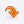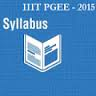UniversitiesLOGINCONTACTMost Recent ArticlesMost Rated ArticlesCategories

Travel/ TourismPGEE
Postgraduate Entrance Examination (PGEE) Pattern & Syllabus
Author : Mammu
 Category : PGEE posted Date :
 Total No.of views : Total No.of Comments : 0
Rating:0 / 5 (0 votes)

Tags :
Postgraduate Entrance Examination (PGEE) Pattern & Syllabus

Postgraduate Entrance Examination (PGEE) Pattern & SyllabusWHAT IS IIITH PGEE?

The Postgraduate Entrance Examination (PGEE) is an all-India examination for admission to PG programmes at International Institute of Information Technology, Hyderabad (IIITH).

IIITH-PGEE Exam Pattern

The entrance examination consists of two papers (No negative marking)

Paper I is General Aptitude

Paper II  is subject paper. Based on the graduation, candidate has to appear for relevant subject papers. The Subjects are

1. Mathematics
2. Computer Science
3. Electronics and Communications Engineering (Objective and Subjective)
4. Structural Engineering (Subjective)
5. Computational Natural Sciences and Bioinformatics
6. Computational Linguistics

The duration for each paper is 1 1/2 hours

IIITH-PGEE Exam Syllabus

PAPER I (GENERAL APTITUDE)

This is objective type question paper and will emphasize on basic aptitude, logical reasoning, basic questions on computers and mathematics.

Note: A minimum cut-off score in this paper is compulsory for evaluation of candidate`s subject paper (Paper II).

PAPER II

MATHEMATICS:

I. Finite Dimensional Linear Vector Space
Linear Independence, Span, Basis, Orthonormal Set, Gram-Schmidt Orthogo- nalization Process, Inner Product, Dual Space, Eigen Space, Rank of a Matrix, Cayley-Hamiltonian Theorem, Similar Matrices, Linear Operator, Hermetian, Unitary and Normal Matrices, Spectral Decomposition.

II. Group, Ring and Field
Basic Concepts of Groups, Cyclic Group, Cosets, Elementary Concepts of Rings and Fields

III. Real Analysis
Concepts of sets of Real numbers, Sequence of Real Numbers, Continuous and Di
?erentiable Functions, Rolle’s Theorem, Mean Value Theorem and Taylor Se- ries, Reimann Integration

IV. Probability Theory
Conditional Probability, Bayes Theorem, Random variable, PDF and CDF, Mo- ment Generating Function, Theoretical Distribution (Binomial, Poisson, Nor- mal, Uniform and Hyper geometric).

V. Complex Analysis
Analytic functions, Integration, Cauchy’s Integral Theorem, Cauchy’s Integral formula, Taylor and Laurent Series, Residue, Contour Integration.

VI. Ordinary Di?erential Equation
Equation of First order and First Degree, Second order Linear Equation with Constant coe
?cients.

VII. Optimization
Linear Programming Problem

COMPUTER SCIENCE

Fundamental Programming Concepts, Control Flow, Functions, Recursion, Basic Data Structures (arrays, lists, stacks, and queues), Basic algorithms (sorting and searching), Boolean Algebra, Digital Building Blocks (AND/OR/NAND/XOR Gates), Karnaugh`s Maps, Computer Organisation, Number Systems. Capability to write programs in C or C++ is expected, Relational Databases (Functional dependencies, SQL), Computer Networks

ELECTRONICS AND COMMUNICATIONS ENGINEERING

Selection for interview for the streams (i) VLSI & Embedded Systems and (ii) Signal Processing and Communication will be based on the score in this paper and the score in the

general aptitude test. This subject examination paper is divided into two parts: Part A (Electronics) and Part B (Signal Processing and Communication)

There will be equal number of questions from Electronics (part-A) and from Signal processing and Communication (part-B). One should attempt questions from both parts in the stipulated time. selection for the interview will be based on the combined score in the two parts.

Part A -  Electronics:

Networks:  Nodal and mesh analysis, Wye-Delta transformation, steady state sinusoidal analysis, time and frequency domain analysis of RLC circuits, Laplace transformations, 2-port networks, Transfer functions.

Electronic Devices:  Theory and Characteristics of Diodes such as Junction and Zener etc, Transistors such as BJT, JFET, and MOSFET etc, Photodiodes.

Analog Circuits: Small signal models and applications of diodes, BJTs, MOSFETs, Biasing of transistors, Types of Amplifiers, Op-amp circuits, Filters, Oscillators, Function generators and wave-shaping circuits.

Digital circuits: Boolean algebra, logic gates, Logic families, combinational, arithmetic, memory and sequential circuits, ADC, Microprocessors and Microcontrollers.

Control Systems: Components of control systems, Open and closed loop control systems, stability analysis, Routh-Hurwitz Criteria, Signal flow graphs, transient and steady state analysis of LTI control systems including frequency response, Root loci, Bode and Nyquist plots, lead and lag compensation, PID control.

Part B - Signal Processing and Communications:

Signals and Systems: Classification of signals, LTI system analysis, System properties, Fourier Series, Fourier Transform, discrete time Fourier series , Laplace Transform, Z-transform, System transfer function, Fourier analysis of LTI systems, Sampling theorem.

Probability theory and random processes: random variables, probability distribution function, probability density function, conditional probability, Bayes theorem, central limit theorem, functions of random variables, random processes, stationary random processes, auto-correlation and cross-correlation functions, power spectrum.

Communication Theory: Analog and Digital Modulation and demodulation, Superheterodyne Receivers, Quantization, Line Coding, Matched filters, Receiver structures in the presence of AWGN, Probability of error.

Digital Signal Processing: Discrete Fourier transform, FFT, Z-transform, Z-domain analysis of LTI systems, Circular convolution, Design of digital IIR and FIR filters.

Electromagnetics: Basics of Electromagnetics, Vector calculus, Maxwell’s equations, Wave equation, Transmission lines, matching networks, S-parameters, Basics of waveguides, optical fibers, and Antennas

STRUCTURAL ENGINEERING

Bending moments and shear forces in beams, stress and strain relations, principal stresses, Mohrs circle, simple bending theory, flexural and shear stresses, torsion, analysis of trusses and frames, analysis of indeterminate structures by force/displacement methods, matrix methods of structural analysis, working and limit state design concepts, design of compression members, beam, slab, footing, staircases, basic concepts of prestressed concrete, riveted and welded joints, steel beam column connections, plate girders and design of base plate.

In addition to this, some questions from engineering mathematics like determinants, matrices, limit, continuity and differentiability, mean value theorems, integral calculus, partial derivatives, maxima and minima, ordinary differential equations and applications, initial and boundary value problems, Laplace and Fourier transforms, test for convergence, sequences and series.

COMPUTATIONAL NATURAL SCIENCES AND BIOINFORMATICS

Selection for interview for the streams (i) Bioinformatics and (ii) Computational Natural Sciences will be based on the score in this paper and the score in the general aptitude test. This examination paper comprises of three sections: Physics, Chemistry, Biology & Bioinformatics, (physics and chemistry only for Post-B.Sc. programmes) and students, depending on their background are expected to attempt any one Section as a major and

another section as a minor part. The questions will be multiple-choice.

PHYSICS SECTION:

Mechanics and General Properties of Matter, Electricity and Magnetism, Kinetic theory and Thermodynamics, Modern Physics, Solid State Physics, Devices and Electronics.

CHEMISTRY SECTION:
Physical Chemistry: Atomic Structure, Theory of Gases, Chemical Thermodynamics, Chemical and Phase Equilibria, Electrochemistry, Chemical Kinetics.

Organic Chemistry :Basic Concepts in Organic Chemistry and Stereochemistry,Aromaticity and Huckel`s rule,Heterocyclic Chemistry, Qualitative Organic Analysis.

Inorganic Chemistry: Periodic Table, Chemical Bonding and Shapes of Compounds, Main Group Elements (s and p blocks), Transition Metals (d block), Analytical Chemistry.

BIOLOGY & BIOINFORMATICS SECTION
Biology: General Biology, Biochemistry and Physiology, Molecular Biology, Cell Biology.

Bioinformatics: Sequence Analysis, Sequence Alignments, Phylogeny, Gene Prediction, Structural Biology.

COMPUTATIONAL LINGUISTICS:

Morphology - Words and how they are formed. What is morphology ? Basic building blocks in morphology - morphemes
Word formation - function based. Other word formation processes - affixation, suffixation, etc. Morphotactics - constraints on affixation, Morpho-phonology, Computational morphology - FSA, paradigms, etc
Word-Classes and part of speech tagging
Lexicography, Syntax, Syntactic structure, Dependency structure, Grammar formalisms
Semantics, Lexical semantics, Sentential semantics
Students should be able to analyse natural language texts in terms
of morphology and basic grammatical structures.